# Eulerian Number

In combinatorics, the Eulerian Number A(n, m), is the number of permutations of the numbers 1 to n in which exactly m elements are greater than previous element.

For example, there are 4 permutations of the number 1 to 3 in which exactly 1 element is greater than the previous elements.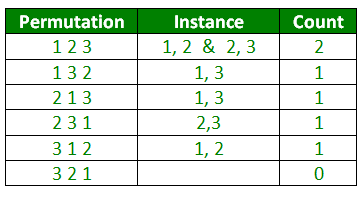Examples :

```Input : n = 3, m = 1
Output : 4
are 4 permutations where 1 no. is
greater.

Input : n = 4, m = 1
Output : 11
```

## Recommended: Please try your approach on {IDE} first, before moving on to the solution.

Eulerian Numbers are the coefficients of the Eulerian polynomials described below.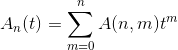The Eulerian polynomials are defined by the exponential generating function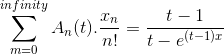The Eulerian polynomials can be computed by the recurrence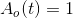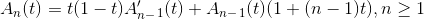An explicit formula for A(n, m) is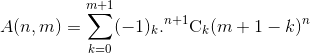We can calculate A(n, m) by recurrence relation: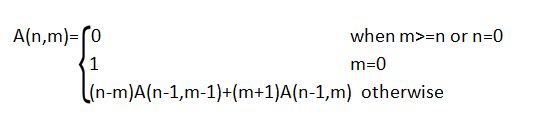Example:
Suppose, n = 3 and m = 1.
Therefore,
A(3, 1)
= (3 – 1) * A(2, 0) + (1 + 1) * A(2, 1)
= 2 * A(2, 0) + 2 * A(2, 1)
= 2 * 1 + 2 * ( (2 – 1) * A(1, 0) + (1 + 1) * A(1, 1))
= 2 + 2 * (1 * 1 + 2 * ((1 – 1) * A(0, 0) + (1 + 1) * A(0, 1))
= 2 + 2 * (1 + 2 * (0 * 1 + 2 * 0)
= 2 + 2 * (1 + 2 * 0)
= 2 + 2 * 1
= 2 + 2
= 4
We can verify this with example shown above.

Below is the implementation of finding A(n, m):

## C++

 `// CPP Program to find Eulerian number A(n, m) ` `#include ` `using` `namespace` `std; ` ` `  `// Return euleriannumber A(n, m) ` `int` `eulerian(``int` `n, ``int` `m) ` `{ ` `    ``if` `(m >= n || n == 0) ` `        ``return` `0; ` ` `  `    ``if` `(m == 0) ` `        ``return` `1; ` ` `  `    ``return` `(n - m) * eulerian(n - 1, m - 1) +  ` `           ``(m + 1) * eulerian(n - 1, m); ` `} ` ` `  `// Driven Program ` `int` `main() ` `{ ` `    ``int` `n = 3, m = 1; ` `    ``cout << eulerian(n, m) << endl; ` `    ``return` `0; ` `} `

## Java

 `// Java rogram to find Eulerian number A(n, m) ` `import` `java.util.*; ` ` `  `class` `Eulerian ` `{ ` `    ``// Return eulerian number A(n, m) ` `    ``public` `static` `int` `eulerian(``int` `n, ``int` `m) ` `    ``{ ` `        ``if` `(m >= n || n == ``0``) ` `            ``return` `0``; ` ` `  `        ``if` `(m == ``0``) ` `            ``return` `1``; ` ` `  `        ``return` `(n - m) * eulerian(n - ``1``, m - ``1``) + ` `            ``(m + ``1``) * eulerian(n - ``1``, m); ` `    ``} ` `     `  `    ``// driver code     ` `    ``public` `static` `void` `main(String[] args) ` `    ``{ ` `        ``int` `n = ``3``, m = ``1``; ` `        ``System.out.print( eulerian(n, m) ); ` `    ``} ` `} ` ` `  `// This code is contributed by rishabh_jain `

## Python3

 `# Python3 Program to find Eulerian number A(n, m) ` ` `  `# Return euleriannumber A(n, m) ` `def` `eulerian(n, m): ` `    ``if` `(m >``=` `n ``or` `n ``=``=` `0``): ` `        ``return` `0``; ` ` `  `    ``if` `(m ``=``=` `0``): ` `        ``return` `1``; ` ` `  `    ``return` `((n ``-` `m) ``*` `eulerian(n ``-` `1``, m ``-` `1``) ``+` `            ``(m ``+` `1``) ``*` `eulerian(n ``-` `1``, m)) ` ` `  `# Driver code ` `n ``=` `3` `m ``=` `1` `print``( eulerian(n, m) ) ` ` `  `# This code is contributed by rishabh_jain `

## C#

 `// C# rogram to find Eulerian number A(n, m) ` `using` `System; ` ` `  `class` `Eulerian { ` `     `  `    ``// Return eulerian number A(n, m) ` `    ``public` `static` `int` `eulerian(``int` `n, ``int` `m) ` `    ``{ ` `        ``if` `(m >= n || n == 0) ` `            ``return` `0; ` ` `  `        ``if` `(m == 0) ` `            ``return` `1; ` ` `  `        ``return` `(n - m) * eulerian(n - 1, m - 1) +  ` `                    ``(m + 1) * eulerian(n - 1, m); ` `    ``} ` ` `  `    ``// driver code ` `    ``public` `static` `void` `Main() ` `    ``{ ` `        ``int` `n = 3, m = 1; ` `        ``Console.WriteLine(eulerian(n, m)); ` `    ``} ` `} ` ` `  `// This code is contributed by vt_m `

## PHP

 `= ``\$n` `|| ``\$n` `== 0) ` `        ``return` `0; ` ` `  `    ``if` `(``\$m` `== 0) ` `        ``return` `1; ` ` `  `    ``return` `(``\$n` `- ``\$m``) * eulerian(``\$n` `- 1, ``\$m` `- 1) +  ` `                 ``(``\$m` `+ 1) * eulerian(``\$n` `- 1, ``\$m``); ` `} ` ` `  `// Driven Code ` `\$n` `= 3; ``\$m` `= 1; ` `echo` `eulerian(``\$n``, ``\$m``); ` ` `  `// This code is contributed by anuj_67. ` `?> `

Output :

```4
```

Below is the implementation of finding A(n, m) using Dynamic Programming:

## C++

 `// CPP Program to find Eulerian number A(n, m) ` `#include ` `using` `namespace` `std; ` ` `  `// Return euleriannumber A(n, m) ` `int` `eulerian(``int` `n, ``int` `m) ` `{ ` `    ``int` `dp[n + 1][m + 1]; ` ` `  `    ``memset``(dp, 0, ``sizeof``(dp)); ` ` `  `    ``// For each row from 1 to n ` `    ``for` `(``int` `i = 1; i <= n; i++) { ` ` `  `        ``// For each column from 0 to m ` `        ``for` `(``int` `j = 0; j <= m; j++) { ` ` `  `            ``// If i is greater than j ` `            ``if` `(i > j) { ` ` `  `                ``// If j is 0, then make that  ` `                ``// state as 1. ` `                ``if` `(j == 0) ` `                    ``dp[i][j] = 1; ` ` `  `                ``// basic recurrence relation. ` `                ``else` `                    ``dp[i][j] = ((i - j) * ` `                     ``dp[i - 1][j - 1]) +  ` `                    ``((j + 1) * dp[i - 1][j]); ` `            ``} ` `        ``} ` `    ``} ` ` `  `    ``return` `dp[n][m]; ` `} ` ` `  `// Driven Program ` `int` `main() ` `{ ` `    ``int` `n = 3, m = 1; ` `    ``cout << eulerian(n, m) << endl; ` `    ``return` `0; ` `} `

## Java

 `// Java rogram to find Eulerian number A(n, m) ` `import` `java.util.*; ` ` `  `class` `Eulerian ` `{ ` `    ``// Return euleriannumber A(n, m) ` `    ``public` `static` `int` `eulerian(``int` `n, ``int` `m) ` `    ``{ ` `        ``int``[][] dp = ``new` `int``[n+``1``][m+``1``]; ` ` `  `        ``// For each row from 1 to n ` `        ``for` `(``int` `i = ``1``; i <= n; i++) { ` `     `  `            ``// For each column from 0 to m ` `            ``for` `(``int` `j = ``0``; j <= m; j++) { ` ` `  `                ``// If i is greater than j ` `                ``if` `(i > j) { ` ` `  `                    ``// If j is 0, then make  ` `                    ``// that state as 1. ` `                    ``if` `(j == ``0``) ` `                        ``dp[i][j] = ``1``; ` ` `  `                    ``// basic recurrence relation. ` `                    ``else` `                        ``dp[i][j] = ((i - j) * ` `                            ``dp[i - ``1``][j - ``1``]) + ` `                        ``((j + ``1``) * dp[i - ``1``][j]); ` `                ``} ` `            ``} ` `        ``} ` ` `  `        ``return` `dp[n][m]; ` `    ``} ` `     `  `    ``// driver code ` `    ``public` `static` `void` `main(String[] args) ` `    ``{ ` `        ``int` `n = ``3``, m = ``1``; ` `        ``System.out.print( eulerian(n, m) ); ` `    ``} ` `} ` ` `  `// This code is contributed by rishabh_jain `

## Python3

 `# Python3 Program to find Eulerian  ` `# number A(n, m) ` ` `  `# Return euleriannumber A(n, m) ` `def` `eulerian(n, m): ` `    ``dp ``=` `[[``0` `for` `x ``in` `range``(m``+``1``)]  ` `             ``for` `y ``in` `range``(n``+``1``)]  ` ` `  `    ``# For each row from 1 to n ` `    ``for` `i ``in` `range``(``1``, n``+``1``): ` ` `  `        ``# For each column from 0 to m ` `        ``for` `j ``in` `range``(``0``, m``+``1``): ` ` `  `            ``# If i is greater than j ` `            ``if` `(i > j): ` `                ``# If j is 0, then make that  ` `                ``# state as 1. ` ` `  `                ``if` `(j ``=``=` `0``): ` `                    ``dp[i][j] ``=` `1` ` `  `                ``# basic recurrence relation. ` `                ``else` `: ` `                    ``dp[i][j] ``=` `(((i ``-` `j) ``*`  `                       ``dp[i ``-` `1``][j ``-` `1``]) ``+`  `                       ``((j ``+` `1``) ``*` `dp[i ``-` `1``][j])) ` `                     `  `    ``return` `dp[n][m] ` ` `  `# Driven Program ` `n ``=` `3` `m ``=` `1` `print``(eulerian(n, m)) ` ` `  `# This code is contributed by Prasad Kshirsagar `

## C#

 `// C# rogram to find Eulerian number A(n, m) ` `using` `System; ` ` `  `class` `Eulerian { ` `     `  `    ``// Return euleriannumber A(n, m) ` `    ``public` `static` `int` `eulerian(``int` `n, ``int` `m) ` `    ``{ ` `        ``int``[, ] dp = ``new` `int``[n + 1, m + 1]; ` ` `  `        ``// For each row from 1 to n ` `        ``for` `(``int` `i = 1; i <= n; i++) { ` ` `  `            ``// For each column from 0 to m ` `            ``for` `(``int` `j = 0; j <= m; j++) { ` ` `  `                ``// If i is greater than j ` `                ``if` `(i > j) { ` ` `  `                    ``// If j is 0, then make ` `                    ``// that state as 1. ` `                    ``if` `(j == 0) ` `                        ``dp[i, j] = 1; ` ` `  `                    ``// basic recurrence relation. ` `                    ``else` `                        ``dp[i, j] = ((i - j) * dp[i - 1, j - 1]) + ` `                                        ``((j + 1) * dp[i - 1, j]); ` `                ``} ` `            ``} ` `        ``} ` ` `  `        ``return` `dp[n, m]; ` `    ``} ` ` `  `    ``// driver code ` `    ``public` `static` `void` `Main() ` `    ``{ ` `        ``int` `n = 3, m = 1; ` `        ``Console.WriteLine(eulerian(n, m)); ` `    ``} ` `} ` ` `  `// This code is contributed by vt_m `

## PHP

 ` ``\$j``)  ` `            ``{  ` ` `  `                ``// If j is 0, then make that  ` `                ``// state as 1.  ` `                ``if` `(``\$j` `== 0)  ` `                    ``\$dp``[``\$i``][``\$j``] = 1;  ` ` `  `                ``// basic recurrence relation.  ` `                ``else` `                    ``\$dp``[``\$i``][``\$j``] = ((``\$i` `- ``\$j``) *  ` `                                    ``\$dp``[``\$i` `- 1][``\$j` `- 1]) +  ` `                                  ``((``\$j` `+ 1) * ``\$dp``[``\$i` `- 1][``\$j``]);  ` `            ``}  ` `        ``}  ` `    ``}  ` ` `  `    ``return` `\$dp``[``\$n``][``\$m``];  ` `}  ` ` `  `// Driver Code ` `\$n` `= 3 ; ` `\$m` `= 1;  ` `echo` `eulerian(``\$n``, ``\$m``) ;  ` ` `  `// This code is contributed by Ryuga ` `?> `

Output :

```4
```

Attention reader! Don’t stop learning now. Get hold of all the important DSA concepts with the DSA Self Paced Course at a student-friendly price and become industry ready.

My Personal Notes arrow_drop_upCheck out this Author's contributed articles.

If you like GeeksforGeeks and would like to contribute, you can also write an article using contribute.geeksforgeeks.org or mail your article to contribute@geeksforgeeks.org. See your article appearing on the GeeksforGeeks main page and help other Geeks.

Please Improve this article if you find anything incorrect by clicking on the "Improve Article" button below.